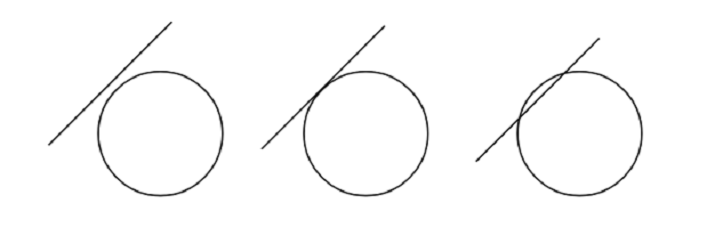# How to Know whether a Line Touches, Intersects or Lie Outside a Circle in Java?

A circle is a closed shape formed by tracing a point that moves in a plane such that its distance from a given point is constant. A line is a straight one-dimensional figure that does not have a thickness, and it extends endlessly in both directions.

In this article we will check if a given line touches a circle or intersects it using java.

We will be given with the centre coordinates and radius of a circle along with equation of the line. We need to check if the given line collides with the circle or not and hence three possible situation arises as mentioned below −

• If a line is outside the circle.

• If a line touches the circle.

• If a line intersects the circle.Now to check the above conditions we will compare the perpendicular distance between center of circle and line with the radius of the circle and name it as “d”.

Now,

• If d > r, then line lie outside the circle.

• If d = r, then line touches the circle.

• If d < r, then line intersect the circle.

We know, equation of the line a*x + b*y + c = 0

“d” can be found using the formula −

$$\mathrm{d=\frac{\rvert\:ax_0\:+\:by_0\:+\:c\:\lvert}{\sqrt{a^{2}\:+\:b^{2}}}}$$

Let’s start!

## To show you some instances

### Instance-1

• Given inputs for the “d” are −

• Radius = 8, center = (0,0), a = 2, b = 5, c = 3.

• After finding the value of “d” the result will be −

• line lies outside the circle.

### Instance-2

• Given inputs for the “d” are −

• Radius = 2, center = (0,0), a = 2, b = 1, c = 8.

• After finding the value of “d” the result will be −

• line lies outside the circle .

### Algorithm

• Step-1 − Declare and initialize the variables.

• Step-2 − Find the distance between center 1 and center 2 of the circle.

• Step-3 − Check for the five conditions of distance.

• Step-4 − Print the result.

## Multiple Approaches

We have provided the solution in different approaches.

• By Using Static Inputs

• By Using User Defined Method

Let’s see the program along with its output one by one.

## Approach-1: By Using Static Input

In this approach, value of radius, center, a, b and c will be assigned to find “d”. Then as per the algorithm we will find if line touches, intersects or lie outside the circle.

### Example

import java.io.*;
public class Main {

//main method
public static void main (String[] args){

//Declaring variables
int a = 2, b = 1, c = 8;

//Finding the distance of line from center of the circle
double dist = (Math.abs(a * 0 + b * 0 + c))/Math.sqrt(a * a + b * b);

// Check for different condition

//print if line touches the circle
System.out.println ( "line touches the circle" );

//print if line intersects the circle
System.out.println( "line intersects the circle") ;
else

//print if line lie outside the circle
System.out.println( "line lie outside the circle") ;
}
}


### Output

line lie outside the circle


## Approach-2: By Using User Defined Method

In this approach, value of radius, center, a, b and c will be assigned to find “d”. Then call a user defined method by passing the given values and as per the algorithm we will find if line touches, intersects or lie outside the circle.

### Example

import java.io.*;
public class Main {

//main method
public static void main (String[] args){

//Declaring variables
int a = 2, b = 5, c = 3;

//calling user defined method
}

//user defined method
static void func(int a, int b, int c, int radius){

//Finding the distance of line from center of the circle
double dist = (Math.abs(a * 0 + b * 0 + c))/Math.sqrt(a * a + b * b);

// Check for different condition

//print if line touches the circle
System.out.println ( "line touches the circle" );

//print if line intersects the circle
System.out.println( "line intersects the circle") ;
else

//print if line lie outside the circle
System.out.println( "line lie outside the circle") ;
}
}


### Output

line intersects the circle


In this article, we explored different approaches to check if a line touches, intersects or lies outside a circle by using Java programming language.

Updated on: 04-May-2023

130 Views